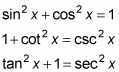##### Trigonometry Workbook For DummiesBecause sine, cosine, and tangent are functions (trig functions), they can be defined as even or odd functions as well. Sine and tangent are both odd functions, and cosine is an even function. In other words,

• sin(–x) = –sin x

• cos(–x) = cos x

• tan(–x) = –tan x

These identities will all make appearances in problems that ask you to simplify an expression, prove an identity, or solve an equation. So what’s the big red flag? The fact that the variable inside the trig function is negative. When tan(–x), for example, appears somewhere in an expression, it should usually be changed to –tan x.

Mostly, you use even/odd identities for graphing purposes, but you may see them in simplifying problems as well. You use an even/odd identity to simplify any expression where –x (or whatever variable you see) is inside the trig function.

The following steps show you how to simplify [1 + sin(–x)][1 – sin(–x)]:

1. Get rid of all the –x values inside the trig functions.

You see two sin(–x) functions, so you replace them both with –sin x to get [1 + (–sin x)][1 – (–sin x)].

2. Simplify the new expression.

First adjust the two negative signs within the parentheses to get (1 – sin x)(1 + sin x), and then FOIL these two binomials to get 1 – sin2 x.

3. Look for any combination of terms that could give you a Pythagorean identity.

Whenever you see a function squared, you should think of the Pythagorean identities. The three Pythagorean identities areLooking at the identities, you see that 1 – sin2 x is the same as cos2 x. Now the expression is fully simplified as cos2 x.